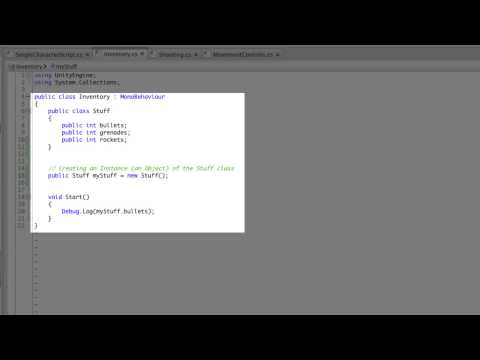# Classes

#### -

How to use Classes to store and organise your information, and how to create constructors to work with parts of your class.## Classes

Principiante Scripting

### Code snippet

``````using UnityEngine;
using System.Collections;

public class SingleCharacterScript : MonoBehaviour
{
public class Stuff
{
public int bullets;
public int rockets;

public Stuff(int bul, int gre, int roc)
{
bullets = bul;
rockets = roc;
}
}

public Stuff myStuff = new Stuff(10, 7, 25);
public float speed;
public float turnSpeed;
public Rigidbody bulletPrefab;
public Transform firePosition;
public float bulletSpeed;

void Update ()
{
Movement();
Shoot();
}

void Movement ()
{
float forwardMovement = Input.GetAxis("Vertical") * speed * Time.deltaTime;
float turnMovement = Input.GetAxis("Horizontal") * turnSpeed * Time.deltaTime;

transform.Translate(Vector3.forward * forwardMovement);
transform.Rotate(Vector3.up * turnMovement);
}

void Shoot ()
{
if(Input.GetButtonDown("Fire1") && myStuff.bullets > 0)
{
Rigidbody bulletInstance = Instantiate(bulletPrefab, firePosition.position, firePosition.rotation) as Rigidbody;
myStuff.bullets--;
}
}
}``````
``````#pragma strict

public class SingleCharacterScript extends MonoBehaviour
{
public class Stuff
{
public var bullets : int;
public var rockets : int;

public function Stuff(bul : int, gre : int, roc : int)
{
bullets = bul;
rockets = roc;
}
}

public var myStuff : Stuff = new Stuff(10, 7, 25);
public var speed : float;
public var turnSpeed : float;
public var bulletPrefab : Rigidbody;
public var firePosition : Transform;
public var bulletSpeed : float;

function Update ()
{
Movement();
Shoot();
}

function Movement ()
{
var forwardMovement : float = Input.GetAxis("Vertical") * speed * Time.deltaTime;
var turnMovement : float = Input.GetAxis("Horizontal") * turnSpeed * Time.deltaTime;

transform.Translate(Vector3.forward * forwardMovement);
transform.Rotate(Vector3.up * turnMovement);
}

function Shoot ()
{
if(Input.GetButtonDown("Fire1") && myStuff.bullets > 0)
{
var bulletInstance : Rigidbody = Instantiate(bulletPrefab, firePosition.position, firePosition.rotation);
myStuff.bullets--;
}
}
}``````
``````import UnityEngine
import System.Collections

public class SingleCharacterScript(MonoBehaviour):

public class Stuff:

public bullets as int

public rockets as int

public def constructor(bul as int, gre as int, roc as int):
bullets = bul
rockets = roc

public myStuff = Stuff(10, 7, 25)

public speed as single

public turnSpeed as single

public bulletPrefab as Rigidbody

public firePosition as Transform

public bulletSpeed as single

private def Update():
Movement()
Shoot()

private def Movement():
forwardMovement as single = ((Input.GetAxis('Vertical') * speed) * Time.deltaTime)
turnMovement as single = ((Input.GetAxis('Horizontal') * turnSpeed) * Time.deltaTime)

transform.Translate((Vector3.forward * forwardMovement))
transform.Rotate((Vector3.up * turnMovement))

private def Shoot():
if Input.GetButtonDown('Fire1') and (myStuff.bullets > 0):
bulletInstance = (Instantiate(bulletPrefab, firePosition.position, firePosition.rotation) as Rigidbody)
myStuff.bullets -= 1``````

### Code snippet

``````using UnityEngine;
using System.Collections;

public class Inventory : MonoBehaviour
{
public class Stuff
{
public int bullets;
public int rockets;
public float fuel;

public Stuff(int bul, int gre, int roc)
{
bullets = bul;
rockets = roc;
}

public Stuff(int bul, float fu)
{
bullets = bul;
fuel = fu;
}

// Constructor
public Stuff ()
{
bullets = 1;
rockets = 1;
}
}

// Creating an Instance (an Object) of the Stuff class
public Stuff myStuff = new Stuff(50, 5, 5);

public Stuff myOtherStuff = new Stuff(50, 1.5f);

void Start()
{
Debug.Log(myStuff.bullets);
}
}``````
``````#pragma strict

public class Inventory extends MonoBehaviour
{
public class Stuff
{
public var bullets : int;
public var rockets : int;
public var fuel : float;

public function Stuff(bul : int, gre : int, roc : int)
{
bullets = bul;
rockets = roc;
}

public function Stuff(bul : int, fu : float)
{
bullets = bul;
fuel = fu;
}

// Constructor
public function Stuff ()
{
bullets = 1;
rockets = 1;
}
}

// Creating an Instance (an Object) of the Stuff class
public var myStuff : Stuff= new Stuff(50, 5, 5);

public var myOtherStuff : Stuff= new Stuff(50, 1.5f);

function Start ()
{
Debug.Log(myStuff.bullets);
}
}``````
``````import UnityEngine
import System.Collections

public class Inventory(MonoBehaviour):

public class Stuff:

public bullets as int

public rockets as int

public fuel as single

public def constructor(bul as int, gre as int, roc as int):
bullets = bul
rockets = roc

public def constructor(bul as int, fu as single):
bullets = bul
fuel = fu

// Constructor
public def constructor():
bullets = 1
rockets = 1

// Creating an Instance (an Object) of the Stuff class
public myStuff = Stuff(50, 5, 5)

public myOtherStuff = Stuff(50, 1.5F)

private def Start():
Debug.Log(myStuff.bullets)``````

### Code snippet

``````using UnityEngine;
using System.Collections;

public class MovementControls : MonoBehaviour
{
public float speed;
public float turnSpeed;

void Update ()
{
Movement();
}

void Movement ()
{
float forwardMovement = Input.GetAxis("Vertical") * speed * Time.deltaTime;
float turnMovement = Input.GetAxis("Horizontal") * turnSpeed * Time.deltaTime;

transform.Translate(Vector3.forward * forwardMovement);
transform.Rotate(Vector3.up * turnMovement);
}
}``````
``````#pragma strict

public var speed : float;
public var turnSpeed : float;

function Update ()
{
Movement();
}

function Movement ()
{
var forwardMovement : float = Input.GetAxis("Vertical") * speed * Time.deltaTime;
var turnMovement : float = Input.GetAxis("Horizontal") * turnSpeed * Time.deltaTime;

transform.Translate(Vector3.forward * forwardMovement);
transform.Rotate(Vector3.up * turnMovement);
}``````
``````import UnityEngine
import System.Collections

public class MovementControls(MonoBehaviour):

public speed as single

public turnSpeed as single

private def Update():
Movement()

private def Movement():
forwardMovement as single = ((Input.GetAxis('Vertical') * speed) * Time.deltaTime)
turnMovement as single = ((Input.GetAxis('Horizontal') * turnSpeed) * Time.deltaTime)

transform.Translate((Vector3.forward * forwardMovement))
transform.Rotate((Vector3.up * turnMovement))``````

### Code snippet

``````using UnityEngine;
using System.Collections;

public class Shooting : MonoBehaviour
{
public Rigidbody bulletPrefab;
public Transform firePosition;
public float bulletSpeed;

private Inventory inventory;

void Awake ()
{
inventory = GetComponent<Inventory>();
}

void Update ()
{
Shoot();
}

void Shoot ()
{
if(Input.GetButtonDown("Fire1") && inventory.myStuff.bullets > 0)
{
Rigidbody bulletInstance = Instantiate(bulletPrefab, firePosition.position, firePosition.rotation) as Rigidbody;
inventory.myStuff.bullets--;
}
}
}``````
``````#pragma strict

public var bulletPrefab : Rigidbody;
public var firePosition : Transform;
public var bulletSpeed : float;

private var inventory : Inventory;

function Awake ()
{
inventory = GetComponent(Inventory);
}

function Update ()
{
Shoot();
}

function Shoot ()
{
if(Input.GetButtonDown("Fire1") && inventory.myStuff.bullets > 0)
{
var bulletInstance : Rigidbody = Instantiate(bulletPrefab, firePosition.position, firePosition.rotation);
inventory.myStuff.bullets--;
}
}``````
``````import UnityEngine
import System.Collections

public class Shooting(MonoBehaviour):

public bulletPrefab as Rigidbody

public firePosition as Transform

public bulletSpeed as single

private inventory as Inventory

private def Awake():
inventory = GetComponent[of Inventory]()

private def Update():
Shoot()``````